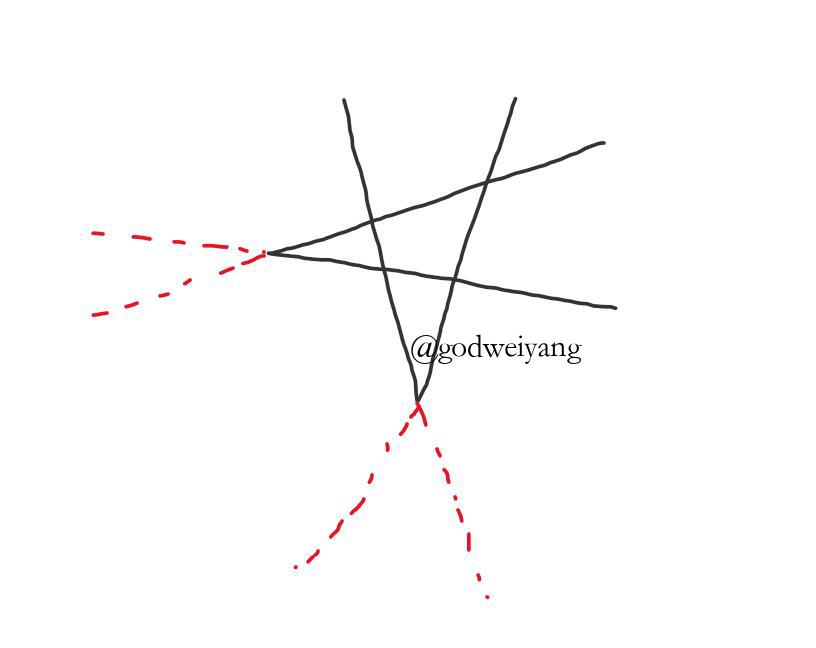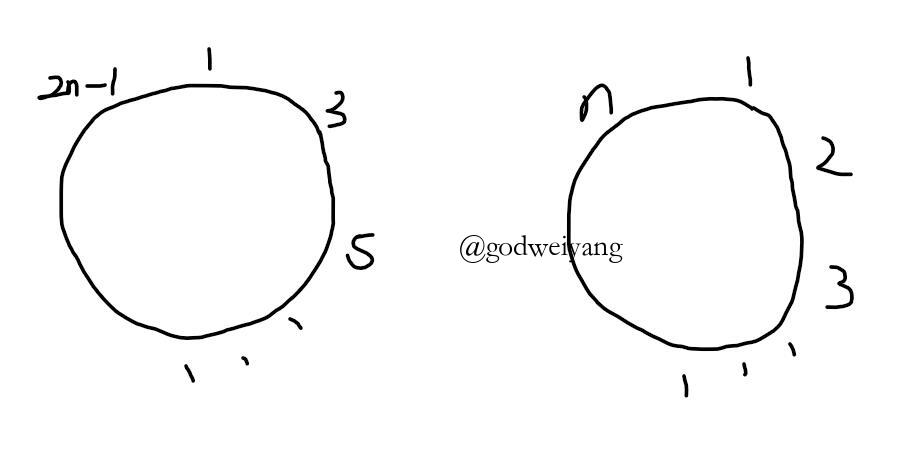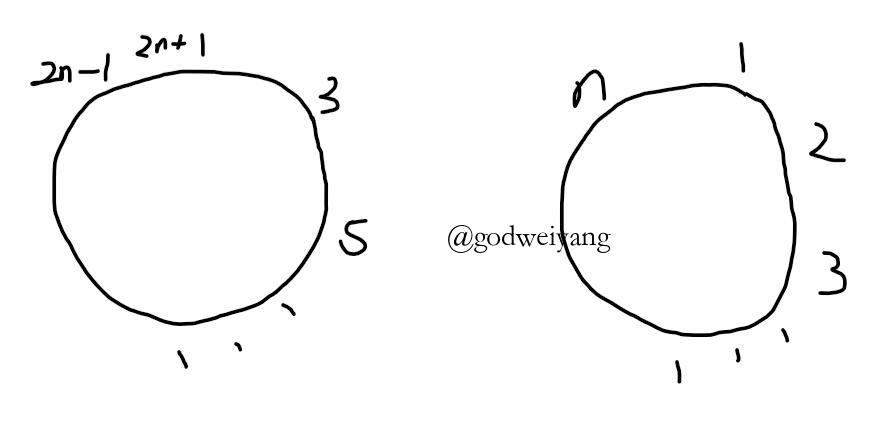# 直线分割平面问题$Z(n)=L(2n)-2n=2n^2-n+1$

# 约瑟夫环问题$J(2n)=2J(n)-1$$J(2n+1)=2J(n)+1$

$J(1)=1$

$J(n)=2l+1$

转载规则

《具体数学-第1课（递归求解实际问题）》 韦阳 采用 知识共享署名 4.0 国际许可协议 进行许可。
上一篇具体数学-第2课（成套方法求解递归式）

2018-03-05毕业论文代码实现思路

2018-02-26
目录